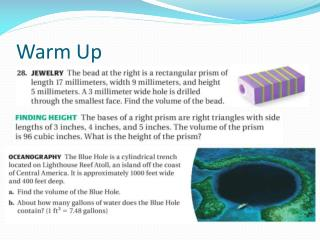# Warm Up - PowerPoint PPT PresentationDownload PresentationWarm Up

Warm Up
Download Presentation## Warm Up

- - - - - - - - - - - - - - - - - - - - - - - - - - - E N D - - - - - - - - - - - - - - - - - - - - - - - - - - -
##### Presentation Transcript

1. Warm Up

2. Discovery • http://www.learner.org/courses/learningmath/measurement/session8/part_b/cylinders.html

3. Formula’s • V = (1/3)Bh • Where B is the area of the base and h is the height

4. 1 a. V = Bh 3 1 1 = ( 4 6)(9) 3 2 EXAMPLE 1 Find the volume of a solid Find the volume of the solid. = 36 m3

5. b. 1 V = Bh 3 1 = (πr2)h 3 1 = (π 2.22)(4.5) 3 EXAMPLE 1 Find the volume of a solid = 7.26π ≈ 22.81 cm3

6. 1 V = bh 3 1 2,226,450 = (x2)(144) 3 EXAMPLE 2 Use volume of a pyramid ALGEBRA Originally, the pyramid had height 144 meters and volume 2,226,450 cubic meters. Find the side length of the square base. SOLUTION Write formula. Substitute.

7. ANSWER Originally, the side length of the base was about 215meters. EXAMPLE 2 Use volume of a pyramid 6,679,350 = 144x2 Multiply each side by 3. 46,384 ≈ x2 Divide each side by 144. 215 ≈ x Find the positive square root.

8. ANSWER 152.42 yd3 for Examples 1 and 2 GUIDED PRACTICE Find the volume of the solid. Round your answer to two decimal places, if necessary. 1. Hexagonal pyramid

9. 2. Right cone ANSWER 163.49m3 for Examples 1 and 2 GUIDED PRACTICE

10. ANSWER The Height of the cone is 12.5m for Examples 1 and 2 GUIDED PRACTICE 3. The volume of a right cone is 1350πcubic meters and the radius is 18meters. Find the height of the cone.

11. Find the volume of the right cone. To find the radius r of the base, use trigonometry. opp. tan 65° = Adj. 16 tan 65° = r 16 r = tan 65° EXAMPLE 3 Use trigonometry to find the volume of a cone SOLUTION Write ratio. Substitute. ≈ 7.46 Solve for r.

12. 1 1 V = (π r 2)h ≈ π(7.462)(16) ≈ 932.45 ft3 3 3 EXAMPLE 3 Use trigonometry to find the volume of a cone Use the formula for the volume of a cone.

13. Find the volume of the solid shown. 1 1 = s3 + Bh 3 3 = 63 + (6)2 6 EXAMPLE 4 Find volume of a composite solid SOLUTION Write formulas. Substitute.

14. ANSWER The volume of the solid is 288cubic meters. EXAMPLE 4 Find volume of a composite solid = 216 + 72 Simplify. = 288 Add.

15. Homework • Pg. 832-833 • 3-25 odd